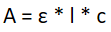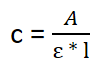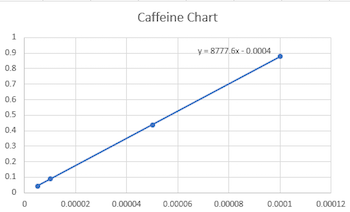# How to Use Spectrophotometry to Understand Beer's Law

An error occurred trying to load this video.

Try refreshing the page, or contact customer support.

Coming up next: What is Centrifugation? - Definition, Process & Uses

### You're on a roll. Keep up the good work!

Replay
Your next lesson will play in 10 seconds
• 0:04 Spectrophotometer
• 1:18 Beer's Law
• 2:44 Determining Molar Absorptivity
• 3:51 Determine Concentration
• 5:47 Lesson Summary
Save Save

Want to watch this again later?

Timeline
Autoplay
Autoplay
Speed Speed Audio mode

#### Recommended Lessons and Courses for You

Lesson Transcript
Instructor: Laura Foist

Laura has a Masters of Science in Food Science and Human Nutrition and has taught college Science.

In this lesson, we'll learn about Beer's Law and how to use spectrophotometry to determine either molar absorbance or concentration in the Beer's Law equation.

## Spectrophotometer

Do you know how much caffeine you drink when you have a cup of coffee? Tea? Soda? You can probably find the answer on the internet, but how was that measured? There are many different methods, but one possible method is using spectrophotometry and Beer's Law.

A spectrophotometer is an instrument that can be used to indirectly determine the amount of a compound present. It works by shining a light onto the sample, then the spectrophotometer measures the amount of light that was absorbed. You first set the spectrophotometer to a specific wave length. For most machines, this is fairly simple using the number pad: you simply type in the desired wavelength. The sample is put into a cuvette. A cuvette is simply a clear, square shaped container. Then the cuvette is put into the spectrophotometer and, after a few seconds, it spits out the results. The results are called absorbance and have no units.

This chosen wave length corresponds to a specific color of light: ultraviolet and infrared lights can also be used, depending on the type of spectrophotometer being used. Each compound will absorb, transmit, and reflect a certain wavelength. If we know what wavelength is absorbed by a certain compound, then we can determine how much of that compound is present by seeing how much of the light was absorbed.

## Beer's Law

So we put the sample into a cuvette and get a number called absorbance. What good does that do? How do we use that information to determine the concentration of the compound of interest in the sample? In order to do this, we use Beer's Law. Beer's Law is that the absorbance, through a known length, is directly proportional to the concentration of the solution. In other words, as long as we know how far the light traveled through the sample, then we can determine the concentration of the solution based on the absorbance. Since we know how long the cuvette is (typically 1 cm), we can determine the concentration.

The equation for Beer's Law is that absorbance equals the molar absorptivity shown as epsilon (in this equation) times the length times the concentration:So there is still one more term we need to define, molar absorptivity. Molar absorptivity is unique for each substance and each wavelength; it refers to how much of a particular wavelength of light will be absorbed by a substance. The units for molar absorptivity is per Molar*cm. In order to determine the molar absorptivity, we run a series of tests with increasing concentrations of the substance, then we graph the results in order to determine epsilon.

Once molar absorptivity has been determined, Beer's Law can be rearranged to solve for concentration once you've determined absorbance, which is, as you can see:## Determining Molar Absorptivity

So, let's look at an example of determining the molar absorptivity. Let's say you want to compare how much caffeine is in coffee and tea. First, you'll get a pure sample of caffeine and make increasing dilutions by mixing increasing amounts of caffeine with water. Let's use 5 μM, 10 μM, 50 μM, and 100 μM. You set the spectrophotometer to 270 nm and you get the following results:

Concentration Absorbance
5 μM 0.0436
10 μM 0.0874
50 μM 0.4381
100 μM 0.8775

Then we chart the results and determine the equation of the chart, as you can see here:To unlock this lesson you must be a Study.com Member.

### Register to view this lesson

Are you a student or a teacher?

#### See for yourself why 30 million people use Study.com

##### Become a Study.com member and start learning now.
Back
What teachers are saying about Study.com

### Earning College Credit

Did you know… We have over 200 college courses that prepare you to earn credit by exam that is accepted by over 1,500 colleges and universities. You can test out of the first two years of college and save thousands off your degree. Anyone can earn credit-by-exam regardless of age or education level.